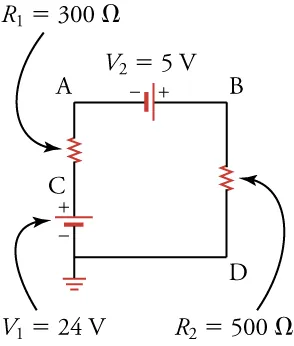Physics

# Critical Thinking Items

PhysicsCritical Thinking Items

### Critical Thinking Items

#### 19.1Ohm's law

11.

An accelerator accelerates He nuclei (change = 2e) to a speed of v = 2 × 106 m/s. What is the current if the linear density of He nuclei is λ = 108 m–1?

1. I = 9.6 × 10–5 A
2. I = 3.2 × 10–5 A
3. I = 12.8 × 10–5 A
4. I = 6.4 × 10–5 A
12 .
How can you verify whether a certain material is ohmic?
1. Make a resistor from this material and measure the current going through this resistor for several different voltages. If the current is proportional to the voltage, then the material is ohmic.
2. Make a resistor from this material and measure the current going through this resistor for several different voltages. If the current is inversely proportional to the voltage, then the material is ohmic.
3. Make a resistor from this material and measure the current going through this resistor for several different voltages. If the current is proportional to the square of the voltage, then the material is ohmic.
4. Make a resistor from this material and measure the current going through this resistor for several different voltages. If the current is inversely proportional to the square of the voltage, then the material is ohmic.

#### 19.2Series Circuits

13.

Given three batteries (5V, 9V, 12V) and five resistors (10, 20, 30, 40, 50Ω) to choose from, what can you choose to form a circuit diagram with a current of 0.175A? You do not need to use all of the components.

1. Batteries (5V, 9V) and resistors (30Ω, 50Ω) connected in series
2. Batteries (5V4, 12V) and resistors (10Ω, 20Ω, 40Ω, and 50Ω) connected in series.
3. Batteries (5V, 9V, and 12V) and resistors (10Ω, 20Ω, and 30Ω) connected in series.
14 .
What is the maximum resistance possible given a resistor of $100$ and a resistor of $40\,\Omega$?
1. $100\,\Omega$
2. $140\,\Omega$
3. $180\,\Omega$
4. $240\,\Omega$
15.

Rank the points A, B, C, and D in the circuit diagram from lowest voltage to highest voltage.1. A, B, C, D
2. B, C, A, D
3. C, B, A, D
4. D, A, B, C

#### 19.3Parallel Circuits

16 .
Can all resistor combinations be reduced to series and parallel combinations?
1. No, all practical resistor circuits cannot be reduced to series and parallel combinations.
2. Yes, all practical resistor circuits can be reduced to series and parallel combinations.
17.

What is the equivalent resistance of the circuit shown below?

Figure 19.23
1. The equivalent resistance of the circuit 14 Ω.
2. The equivalent resistance of the circuit 16.7 Ω.
3. The equivalent resistance of the circuit 140 Ω.
4. The equivalent resistance of the circuit 195 Ω.

#### 19.4Electric Power

18.

Two lamps have different resistances. (a) If the lamps are connected in parallel, which one is brighter, the lamp with greater resistance or the lamp with less resistance? (b) If the lamps are connected in series, which one is brighter? Note that the brighter lamp dissipates more power.

1. (a) lamp with greater resistance; (b) lamp with less resistance
2. (a) lamp with greater resistance; (b) lamp with greater resistance
3. (a) lamp with less resistance; (b) lamp with less resistance
4. (a) lamp with less resistance; (b) lamp with greater resistance
19.

To measure the power consumed by your laptop computer, you place an ammeter (a device that measures electric current) in series with its DC power supply. When the screen is off, the computer draws 0.40 A of current.
When the screen is on at full brightness, it draws 0.90 A of current. Knowing the DC power supply delivers 16 V, how much power is used by the screen?

1. The power used by the screen is −8.0 W.
2. The power used by the screen is 0.3 W.
3. The power used by the screen is 3.2 W.
4. The power used by the screen is 8.0 W.
Order a print copy

As an Amazon Associate we earn from qualifying purchases.